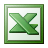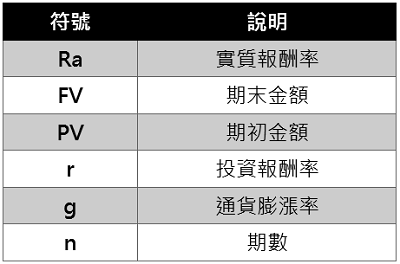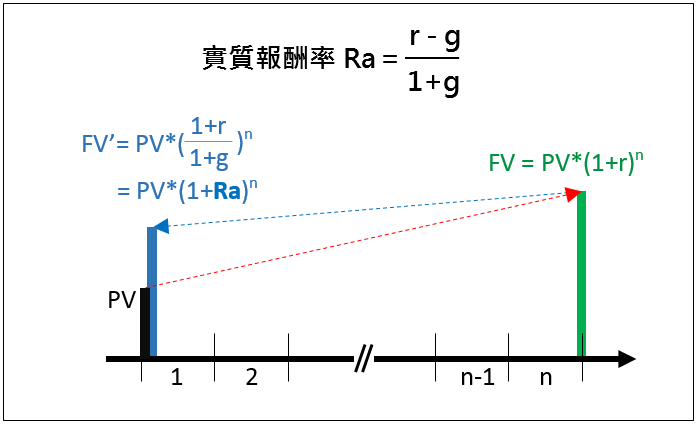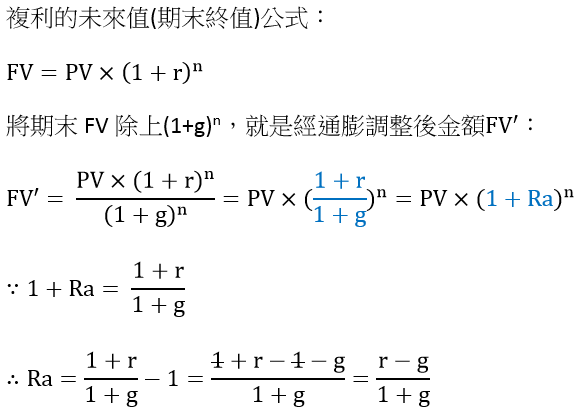# 通膨調整之實質報酬率

﻿

12%的投資報酬率扣掉2%的通貨膨漲率，應該是10%。而精確計算出來的實值報酬率卻是9.804%，是將10%除上(1+2%)得到的結果。其實，通膨調整後的實質報酬率公式如下所示：

=500000*(1 + 9.804%)30
= 827萬元整

【=500000*(1+9.804%)^30】

## 費用經通膨調整後之現值

=300000/(1+4.902%)12
=16萬8,934元 【=300000/(1+4.902%)^12】

=300000*(1/(1+4.901961%)12 + 1/(1+4.901961%)13+1/(1+4.901961%)14+1/(1+4.901961%)15)
=62萬9,831
【=300000*(1/(1+4.901961%)^12+ 1/(1+4.901961%)^13+1/(1+4.901961%)^14+1/(1+4.901961%)^15)】

### 分期籌措教育基金

62萬9,828是一筆不小的金額，也可以透過分期來籌措。如果以15年來分攤，投資報酬率一樣為7%，那麼每月得定期定額多少金額？

=PMT(月報酬率, 年數*12, -教育準備金現值)   ， 月報酬率=NOMINAL(年報酬率, 12)/12
=PMT(NOMINAL(7%, 12)/12, 15*12,-629831)
=5,585.6元## 退休準備金之計算=600000/(1+5%)0+ 612000/(1+5%)1+ 624240/(1+5%)2+…….+ 909400/(1+5%)21
=990萬1,648元

=PV(實質年利率, 年數, -每年費用現值, 0, 1)
=PV((5%-2%)/(1+2%), 22, -600000, 0 , 1)
=990萬1,648

### 驗算=PV((5%-2%)/(1+2%), 40, -600000, 0 , 1)
=1,441萬3,508元

## 實質報酬率公式﻿

■ 本網站內容儘可能精確完整，但不保證無誤。若做為投資依據，風險請自行斟酌 ，本網站不負賠償之責任。
■ 網站所有資料均為版權所有，非經書面允許請勿轉載或使用。